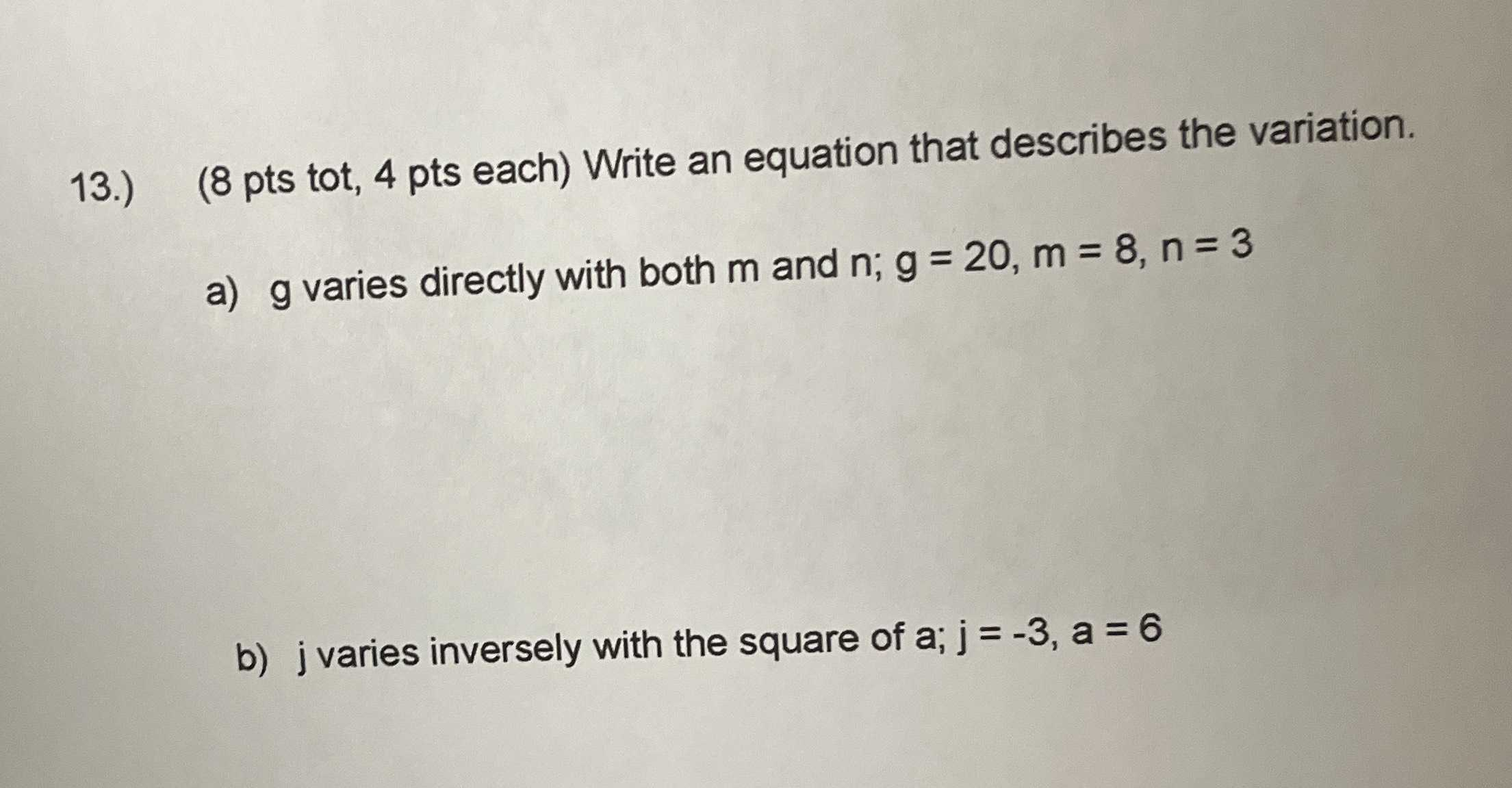### ¿Todavía tienes preguntas de matemáticas?

Pregunte a nuestros tutores expertos
Algebra
Pregunta13.) (8 pts tot, $$4$$ pts each) Write an equation that describes the variation.

a) $$g$$ varies directly with both $$m$$ and $$n ; g = 20 , m = 8 , n = 3$$

b) j varies inversely with the square of $$a ; j = - 3 , a = 6$$

$$a) g = \frac{5}{6} mn$$
b) $$j = \frac{- 108 }{a^2}$$